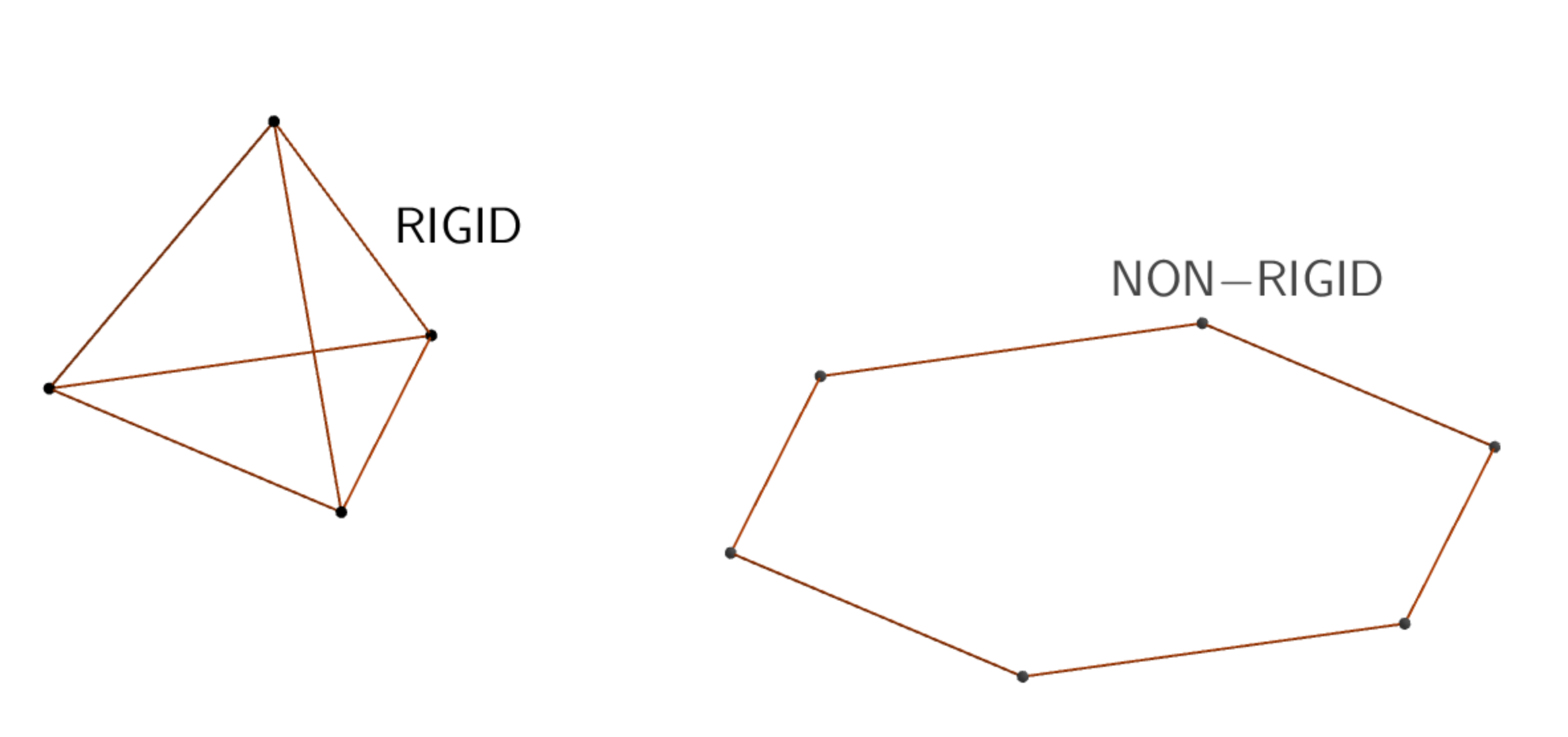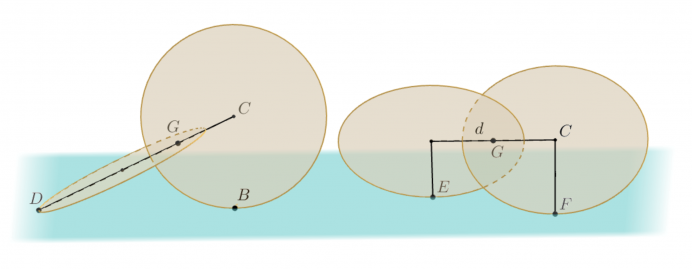###This week, the Varsity Math team is visiting the Architecture School to lend a helping hand in the design of some of the more experimental building concepts.Hex Truss | Junior Varsity
Tamara, one of the architecture students, wants to design the superstructure of the building for her senior project in a novel way. Her idea is to use rigid beams connected by flexible joints. All of the beams are the same length, and the size of the joints is negligible compared to the length of the beams. The joints allow the beams to rotate freely with respect to one another—they create no constraint on the angle or orientation among any of the beams. Nevertheless, she’s discovered it is possible to create rigid structures with this system, such as a regular triangular pyramid, consisting of six beams and four joints (see the diagram).

Tamara wants the base of her building to consist of a regular hexagon. Unfortunately, a hexagon made from this system is not at all rigid, so she needs to add more beams and joints to brace her hexagon and make the overall superstructure rigid. All of the beams and joints must lie at the level of the hexagon or above it; she can’t have any portion of the superstructure extend below the hexagon. Naturally, Tamara would also like to be as efficient and cost effective as possible.

What is the minimum number of beams Tamara can use (not counting the six in the hexagon) to brace the hexagon and create a rigid superstructure for her building?

[asciimathsf]

Windows on the World | Junior Varsity Relay
Another student, Nyman, is designing a perfectly spherical concept building for his project. The building will have identical circular windows embedded in the surface of the sphere. As it happens, the number of windows in the design equals the solution of last week’s Cross Training problem. Nyman feels that the perfect balance and order for his design is achieved when the minimum distance between any pair of windows in his building is as large as possible. Also, for reasons nobody in the department quite understands, Nyman wants the radius of his building to be precisely `sqrt {8 + 2 sqrt 2}` meters.

How far apart in meters, center to center, will the closest pair of windows in Nyman’s building be? Measure this distance as the straight line between the centers (which can go through the interior of the spherical building), not by the distance along the surface of the building.

### Solutions to Week 6

Cross Training.  The answer to this problem is needed for the relay this week, so we’re not giving it away yet.

Sesquicycle.  First, we need to know where the center of gravity of the sesquicycle is. In finding centers of gravity, you can always replace any portion of an object with a point mass at the center of gravity of that portion. The center of gravity of a disk is at its center, so the problem reduces to finding the center of gravity of two equal masses at the centers of the two disks. That’s clearly at the midpoint of the line segment joining the two centers. So the center of gravity of the sesquicycle is midway between the centers of the disks.There are two special configurations, shown in the above diagram, in which it is relatively easy to calculate the height of this center of gravity. In the position on the left, one disk is perpendicular to the ground; and in the other position, both disks are inclined at 45 degrees and the line connecting the two centers is parallel to the ground. So the plan for solution is to calculate the height of the center of gravity in these two configurations as a function of the separation of the disk centers, and see if at some separation they become equal. If so, that must be the required separation.So suppose the centers of the two disks are separated by d meters. In the first configuration, the point D on one disk diametrically opposite the center C of the other is in contact with the ground. Seen from the side (see right), a right triangle is formed by D, C and B, the point a which the circle centered at C contacts the ground. (It must be a right triangle because any tangent to a circle is perpendicular to the radius which meets the point of tangency.) Therefore, in this configuration, the center C is one radius, or ¼ meter, above the ground. Using similar triangles, the height h of the center of gravity G is to ¼ as the distance from D to G is to the distance from D to C. Writing this as an equation,

h / ¼ = (¼ + d/2) / (¼ + d) = (1 + 2d) / (1 + 4d).

Dividing both sides by 4, we have h = (1 + 2d) / 4(1 + 4d).In the other configuration, the sesquicycle has rotated 45 degrees, so that the line segment joining the two centers is parallel to the ground, and the radius from the center of each circle to the point of contact of that circle with the ground is perpendicular to the line joining the two centers. Looked at end-on (see left), in the direction of line joining the two centers, you can see that the center of gravity G (which is on that line joining the two circle centers) is at the apex of an isosceles right triangle with legs equal to ¼ meter and hypotenuse along the ground. In other words, the height of G is now half the diagonal of a square of side ¼, which is `sqrt 2 // 8`.

Setting the heights in the two configurations equal, `(1 + 2d) // 4(1 + 4d) = sqrt 2 // 8`. Cross multiplying, `8(1 + 2d) = 4 sqrt 2(1 + 4d)`. Dividing by four and solving for `d` yields

`d = (2  – sqrt 2) // (4 sqrt 2  – 4) = sqrt 2 (sqrt 2  – 1) // 4(sqrt 2  – 1) = sqrt 2 // 4`.

So you should recommend that your teammate separate the centers of the sesquicyle disks by `bb{sqrt 2 // 4}` meters.

Bonus Answer. For those of you who have been waiting patiently to see how those trees in the Carambola Orchard were arranged, here’s a picture. The category 3 trees are shown with smaller dots, the category 5 trees with larger ones.### Previous Weeks

Week 6: Cross Training & Sesquicycle, solutions to Week 5 and Relay 1
Week 5: Laborious Lockup & Prime Event, solutions to Week 4
Week 4: Unsustainable Agriculture & Save the Camel, solutions to Week 3
Week 3: Deranged Assistant & Rush Job, solutions to Week 2
Week 2: Carambola Orchard & Farm Roundup, solutions to Week 1
Week 1: Instant Interrogation & Urn Respect

Find more puzzles at varsity.momath.org.